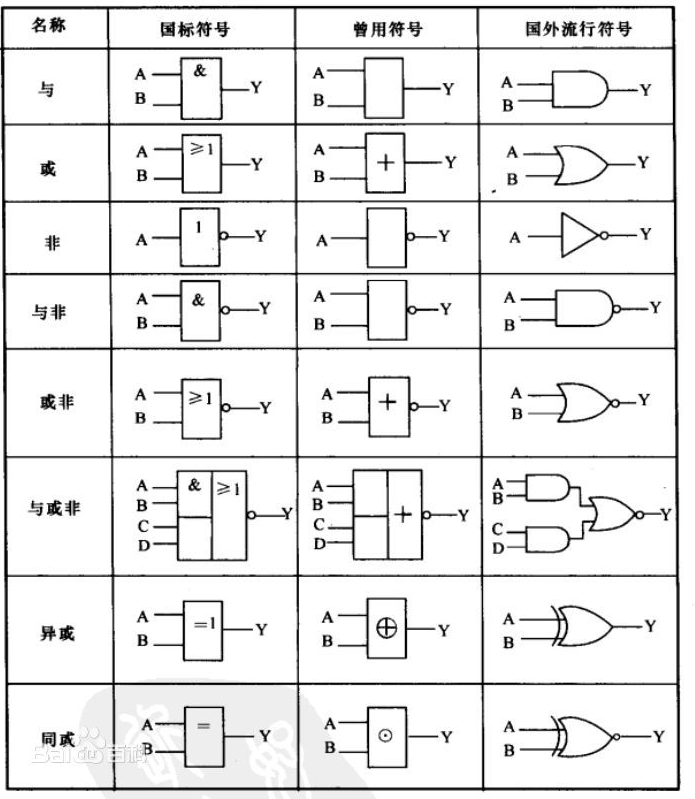## 3.逻辑器件及其功能

eg: 1&1=1 1&0=0 0&1=0 0&0=0

eg:1|1=1 1|0=1 0|1=1 0|0=0

eg:1! =0      0! =1